# Undestanding math notation

Hi all,
I’m trying to implement the layer below: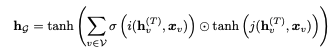However, I’m having trouble understanding the math notation. My understanding is that we first compute the function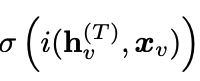Then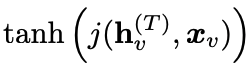Followed by the element-wise multiplication: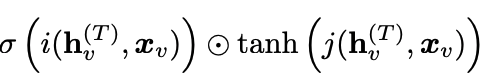The problem is I’m not sure where the summation is happening - if it is on the element-wise product: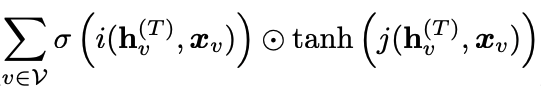or just in the output of function i(hv, xv)

2 Likes

I am not sure what this is for, but basically you have this variable v and you are computing and summing the elementwise product for all possible values of v

2 Likes

Try this

1 Like

Let’s try to break this down together. Here’s the equation(that I typed in using Latex markup, hopefully copied correctly)

h_g = tanh \left( \sum_{v \in V} \sigma \left( i(h_v^{(T)}, x_v) \right) \odot tanh \left( j(h_v^{(T)}, x_v) \right) \right)

I’m rather rusty at reading heavy math equations these days(uni. long time ago), so I’ll try my best. Please do correct my if my understanding is wrong.

In plain english,

• the tanh operation
• on the result of
• the summation of
• ⊙ operation on (assuming this is elementwise multiplication like you said)
• two tensors (indexed with v)
• the summation ranging on elements in V indexed with v

The above doesn’t really feel intuitive, so I think it’s better to start from the inside and walk outside of the equation.

There’s two tensors at the innermost part, both of these have elements indexed with v. Since the summation ranges over V(indexed with v), you can get rid of the confusion that summation only applies to the first tensor. It’s applied to the result of the ⊙ operation(else the v in second portion wouldn’t make sense).

So, basically you have the following two tensors (v being in range of V).
Assume the A, B only for simplification. I do not know if this representation would be mathematically correct.

A_v = \sigma \left( i(h_v^{(T)}, x_v) \right)

and

B_v = tanh \left( j(h_v^{(T)}, x_v) \right)

Then you perform the ⊙ operation between them, sum it up(over the vs) and perform tanh on it.

h_g = tanh \left( \sum_{v \in V} A_v \odot B_v \right)

(Of course, in Pytorch that’s not the order of execution for the code, I only wrote it down here in the inside → out format to help motivate the explanation)

Hopefully, that helps to some extent.
And even more hopefully, this explanation is correct.11 Likes

thank so much for the detailed explanation!

1 Like

@suvash just wanted to let you know – I’ve seen you explain things on the forums a few times, and every time (including this one) you’ve done a really amazing job of being clear and understandable. Explanations are not easy!

11 Likes

Thank you Jeremy !1 Like
1 Like

It would be nice to write all that expression down as vectorized code: starting with the outside, memberwise multiply and sum is just… Dot product:

h_g = tanh({ \sum_{v \in V} A_v \odot B_v }) = tanh (\overline{A} \cdot \overline{B})

So in Pytorch terms it will be:

h_g  = nn.Tanh(A@B)


Of course I’m assuming that you’ve a proper way to compute A and B.
BTW, written like this is way less scary6 Likes

Thank you all for the great explanations! The papers I’m trying to reproduce are quite heavy for my level of math but I’m making some progress thanks to you!

I’m guessing you are trying to code LSTM from scratchChapter 12 of the fastai book, under section Build LSTM from Scratch could provide some help if you should need any.